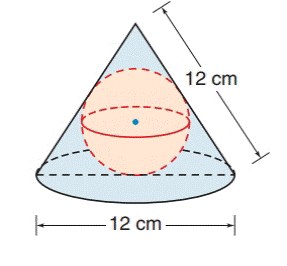Chapter 9.4, Problem 30E### Elementary Geometry for College St...

6th Edition
Daniel C. Alexander + 1 other
ISBN: 9781285195698

#### Solutions

Chapter
Section### Elementary Geometry for College St...

6th Edition
Daniel C. Alexander + 1 other
ISBN: 9781285195698
Textbook Problem
1 views

# A sphere is inscribed in a right circular cone whose slant height has a length equal to that of the diameter of its base. What is the length of the radius of the sphere if the slant height and the diameter of the cone both measure 12 cm?To determine

To find:

The length of the radius of the sphere, inscribed in a right circular cone.

Explanation

Approach:

A sphere is a three dimensional solid figure, which is made up of all the points in space, which lie at a common distance, called the radius, from a fixed point called the center of the sphere.

The surface area of a sphere S =4πr2, where r is the radius of the sphere.

Volume of a sphere V = 43πr3.

For right circular cone of base radius (r), slant height (l) and altitude (h),

Total surface area of the cone = πrl+r.

Curved or lateral surface area of the cone = πrl.

Volume of the cone = 13πr2h.

The Pythagorean theorem for a right angle triangle states that the square of the hypotenuse is equal to the sum of the squares of the other two sides. The longest side of the right angle triangle is called the hypotenuse.

Calculation:

Consider a sphere inscribed in a right circular cone. The slant height of the cone has length equal to the diameter of its base.

For right circular cone of base radius (r), slant height (l) and altitude (h), and radius of sphere = (R).

slant height of cone = 12 cm = (l)

diameter of the cone = 12 cm = (d)

radius of cone = (r) = 6 cm

By using Pythagorean Theorem,

h2+r2=l2

h2+(6)2=(12)2

h2=144-36

h2=108

h=63

In the above figure

BC- = r, radius of the base of the cone

### Still sussing out bartleby?

Check out a sample textbook solution.

See a sample solution

#### The Solution to Your Study Problems

Bartleby provides explanations to thousands of textbook problems written by our experts, many with advanced degrees!

Get Started

#### Evaluate the expression sin Exercises 116. 52535252

Finite Mathematics and Applied Calculus (MindTap Course List)

#### In Exercises 6372, evaluate the expression. 70. | 6| 3

Applied Calculus for the Managerial, Life, and Social Sciences: A Brief Approach

#### Convert from radians to degrees. 10. 83

Single Variable Calculus: Early Transcendentals

#### The third partial sum of is:

Study Guide for Stewart's Single Variable Calculus: Early Transcendentals, 8th